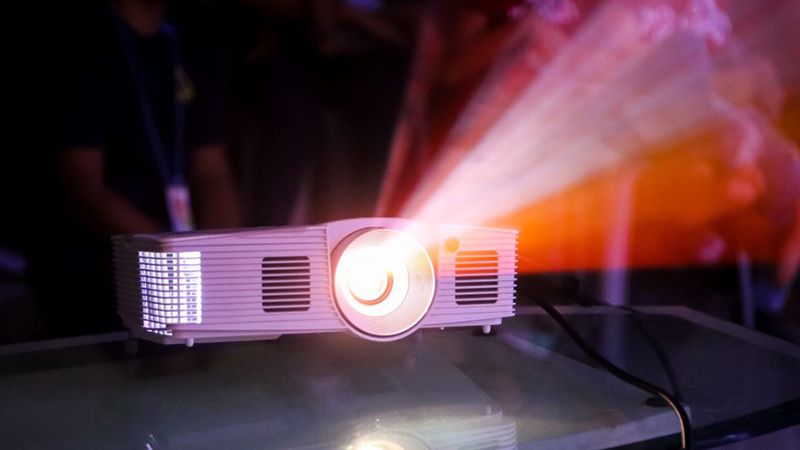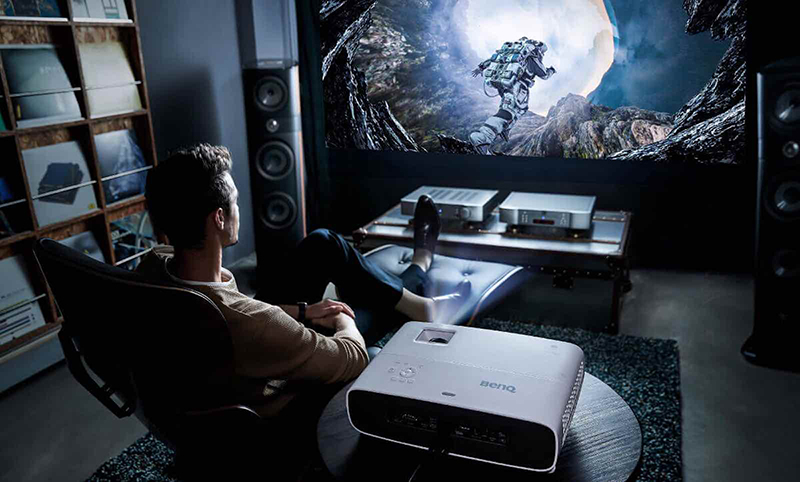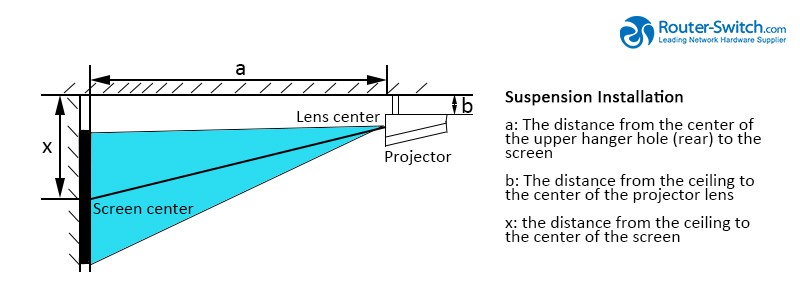# Buyer Guide: How to Calculate the Installation Distance and Size of the Projector Screen?Now projectors are more and more necessary and convenient for enterprise or consumer. There are many brands of projectors in the market, such as Xiaomi Projectors , XGIMI Projectors , JMGO Projectors , Dangbei Projectors , etc.

No matter where the projector is arranged, the influence of the projection distance on the screen size cannot be ignored. Since each projector has a different throw ratio, be sure to read the manual carefully before installing the projector, and install the projector according to the projection distance comparison table in the manual, or calculate it yourself according to the projection ratio parameter. The following is an introduction to the most practical calculation method for the installation distance of the projector.The specific calculation formula is as follows:

1. If the projector screen is 16:9, the formula is:

Projection distance = screen size X 0.87 X 0.0254 X projector’s aspect ratio.

1. If the projector screen is 4:3, the formula is:

Projection distance = screen size X 0.8 X 0.0254 X projector’s aspect ratio.

1. If the projector screen is 16:10, the formula is:

Projection distance = screen size X 0.848 X 0.0254 X projector’s aspect ratio.

1. Calculation example:

Take the 16:9 wide screen as an example

Projection distance = screen size X 0.87 X 0.0254 X projector’s aspect ratio.Conversely, we can get another formula:

The size of the screen = projection distance / (0.87 X 0.0254 X projection ratio).

With the above formula, the following calculations can be made:

You can quickly figure out how far and how big the screen should be. For example, if you want to cast 2 meters away, the projection ratio of the projector is 1.2, and what size should the smallest screen be?

The size of the screen = 2 / (0.87X0.0254X1.2) = 75 inches.

Of course, the projection distance can also be calculated according to the screen size. The size of the screen I bought is 80 inches, and the projector’s aspect ratio is 1.2, so what is the projection distance?

Projection distance=80 X 0.87 X 0.0254 X 1.2=2.12 meters.

Of course, you can quickly figure out what space is equipped with what aspect ratio projector? For example, if you want to achieve a 100-inch screen at a distance of 2.5 meters, what kind of projector to choose?

Projector’s aspect ratio =2.5 / (100 X 0.87 X 0.0254)=1.13

The above is a detailed calculation method for the installation distance of the projector. The following is a summary table of common screen sizes and projection distances.Screen size: Visual range, Diagonal measurement (Inch)                                                                                                                               (mm)

 Screen size 40 60 80 100 120 150 180 200 250 300 a Max 1244 1842 2400 3038 3635 4532 5429 6027 7521 9061 Min 1431 2121 2812 3502 4193 5228 6464 6954 8680 10406 b b+240 b+342 b+443 b+545 b+646 b+799 b+954 b+1053 b+1307 b+1563

Related Topics:

Buyer Guide: 5 Tips You Must Know When Choosing Projectors

Buyer Guide: How to Select Storages for the Enterprise?

Buyer Guide: Choose Cisco ISR Featured Routers, ISR4000, ISR1000, and More

Buyer Guide: 5 Tips When Choosing IP Phones

Buyer Guide: 4 Misunderstandings when choosing an Access Point